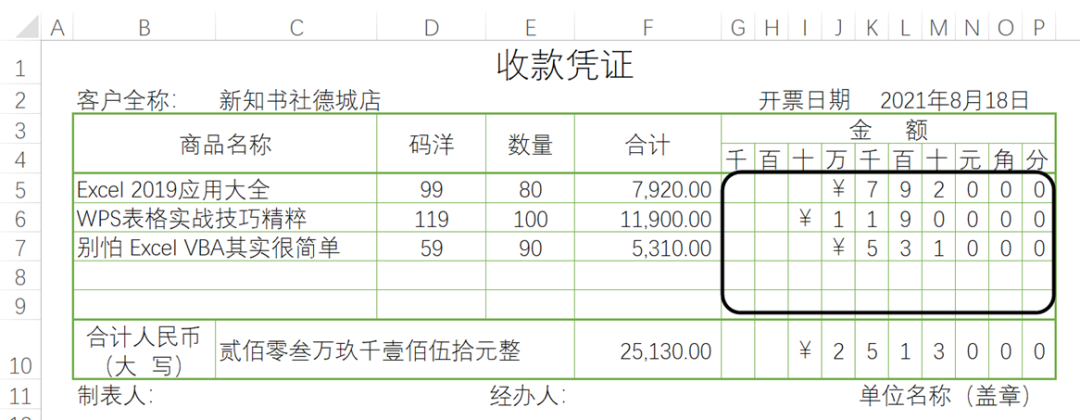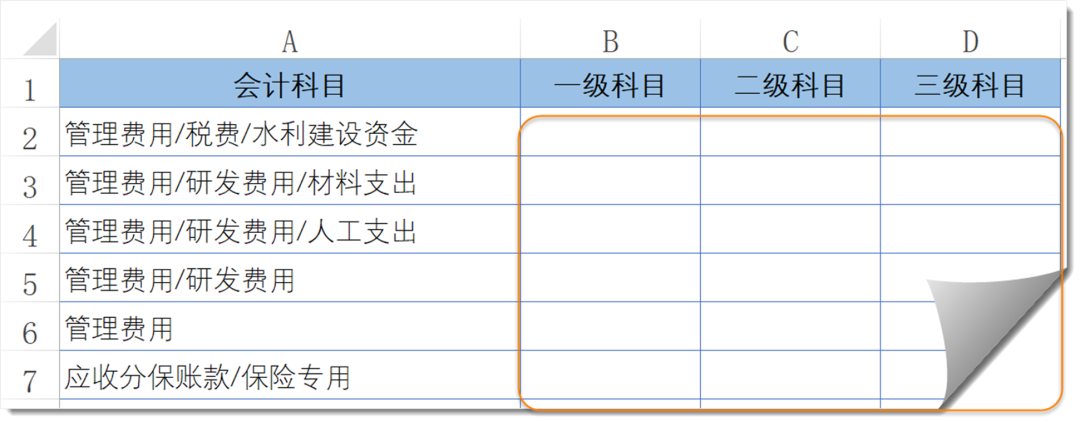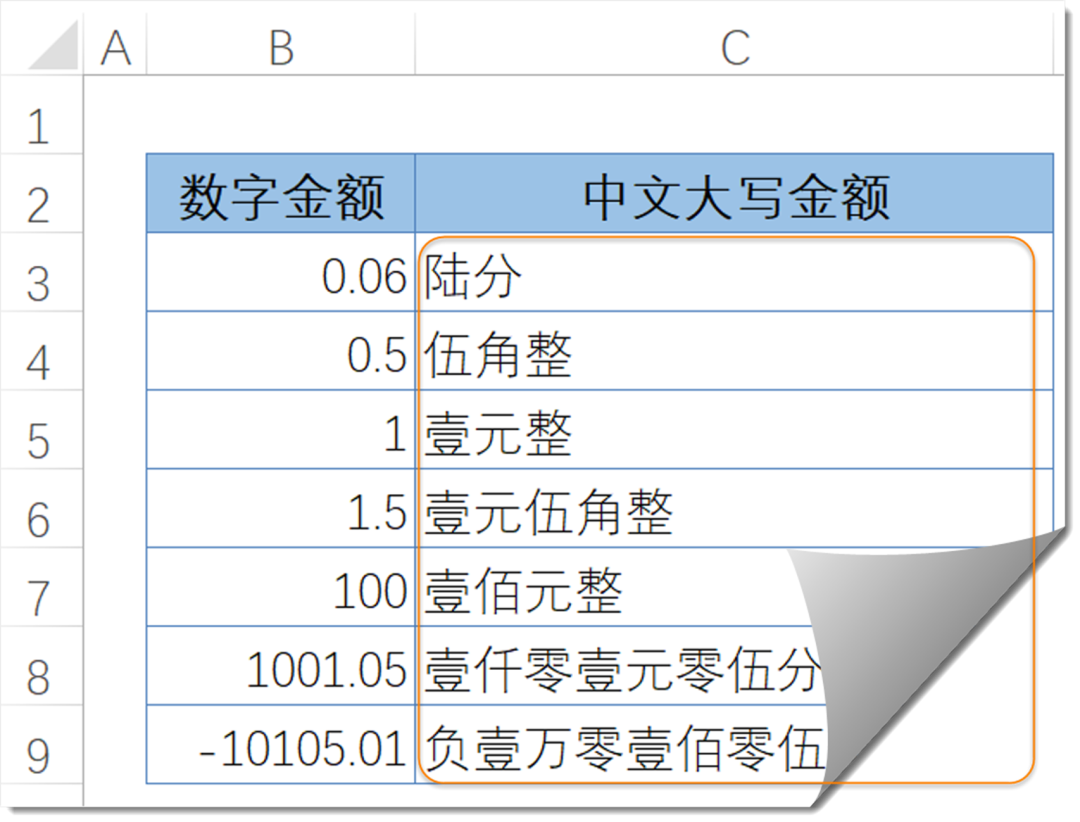# 几个模式化的公式用法，收藏备用

2022-07-16 10:20:55 excel教程

## 1、分列填写凭证金额`=IF(\$F5,LEFT(RIGHT(" ￥"&\$F5/1%,COLUMNS(G:\$P))),"")`

## 2、拆分会计科目B2单元格输入以下公式，将公式向右向下复制。
`=TRIM(MID(SUBSTITUTE(\$A2,"/",REPT(" ",99)),COLUMN(A1)*99-98,99))`

## 3、转换中文大写金额C3单元格输入以下公式，将公式向下复制。

`=SUBSTITUTE(SUBSTITUTE(SUBSTITUTE(IF(B3<0,"负","")&TEXT(INT(ABS(B3)),"[dbnum2];; ")&TEXT(MOD(ABS(B3)*100,100),"[>9][dbnum2]元0角0分;[=0]元整;[dbnum2]元零0分"),"零分","整")," 元零",)," 元",)`